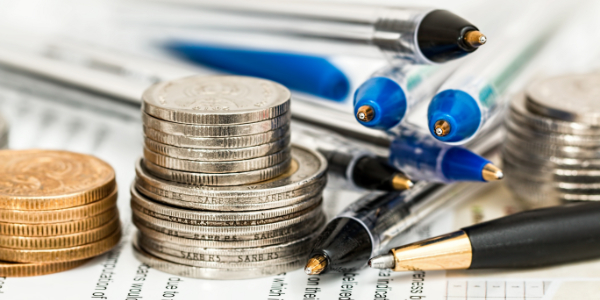Home / Money Blog

## What if I get paid off early?Note: You can use any financial calculator to do this problem, but if you want the BEST, you can get our 10bii Financial Calculator for iOS, Android, Mac, and Windows!Image Source
THE SCENARIO Last time, we talked about what would happen if I sold a note after owning it for a relatively short period of time. However, nothing's forcing me to sell the note. So what happens if I hold onto it, and the borrower pays it off early (through a refinance or just by coming up with a bunch of cash) rather than paying it off entirely over time? The question: I bought a fully-amortizing note for \$79,000 with an unpaid balance of \$100,000, a payment of \$898.83, and remaining term of 15 years. I collect payments for 6 years, and the borrower pays it off. What's my return?
THE SOLUTION This one has several parts.
1. Figure out the interest rate the borrower is paying.
2. Figure out how much the borrower still owes after 6 years.
3. Figure out my rate of return.
First things first, make sure the calculator is using 12 Payments per Year. Step 1: The borrower's rate N: 180 (The note has 15 x 12 = 180 months left) I/YR: (This is what I'm trying to find) PV: 100,000 (The borrower starts out owing \$100,000) PMT: -898.83 (The borrower's monthly payment is \$898.83) FV: 0 (The loan amortizes fully)

The borrower's interest rate is 7.00%.

Step 2: The payoff amount N: 72 (The borrower pays off in 6 x 12 = 72 months) I/YR: 7.00 (The loan's interest rate is 7.00%, calculated in Step 1) PV: 100,000 (The borrower starts out owing \$100,000) PMT: -898.83 (The borrower's monthly payment is \$898.83) FV: (This is what I'm trying to find)

After 6 years, the borrower still owes \$71,870.02, which is the payoff amount.

Note that if you used the calculated result from Step 1 here instead of typing it in, you'd be using 7.000030929%, and your FV would be \$0.20 higher than this. Since banks don't tend to use 6+ decimal points in their interest rates, I used a flat 7.00%, as I feel that's probably what would happen in the real world in this situation. On a side note, if a difference of 20 cents will make or break a deal, you might want to think twice about doing the deal. Step 3: Calculating my return N: 72 (I get paid off after 6 x 12 = 72 months) I/YR: (This is what I'm trying to find) PV: -79,000 (I paid \$79,000 for the note) PMT: 898.83 (I received \$898.83 monthly payments from the borrower) FV: 71,870.02 (The borrower pays off the loan for \$71,870.02, calculated in Step 2)

My return on my \$79,000 investment is 12.64%.

If you recall from last time, I was able to get an even higher return by selling the note to Brenda, but the reason for the difference was the shorter length of time I held the note - if you were to run this same question but with the 3-year time frame from last time, you'd see that my returns would be higher than 12.64%... and even higher than when I sold the note to Brenda.

What do you think? Would you prefer to get paid off, or would you like to continue receiving payments? Would you be happy getting a big payoff for a higher return, or do you favor the income? Why or why not? Let us know in the comments!